# How to remove a specific element from Queue

• Last Updated : 29 Sep, 2021

Given a queue q[] and an integer K, the task is to define a method to remove a specific element from the queue q[]. If there are multiple occurrences of element K, then, remove the first one from the queue q[].

Examples:

Input: q[] = {10, 20, 30, 40, 50, 60}, K = 30
Output: {10, 20, 40, 50, 60}
Explanation: After removal of 30, the queue becomes {10, 20, 40, 50, 60}.

Input: q[] = {1, 2, 3, 3}, K = 3
Output: {1, 2, 3}
Explanation: After removal of the first occurrence of 3, the queue becomes {1, 2,  3}.

Approach: The idea is to create a temporary queue ref[] and store all the elements in it, until K is found. Then, remove K from the original queue q[], and insert the remaining elements back into the queue q[]. Follow the steps below to solve the problem: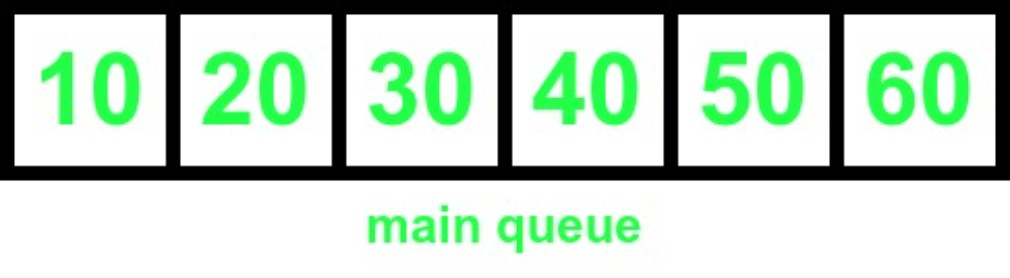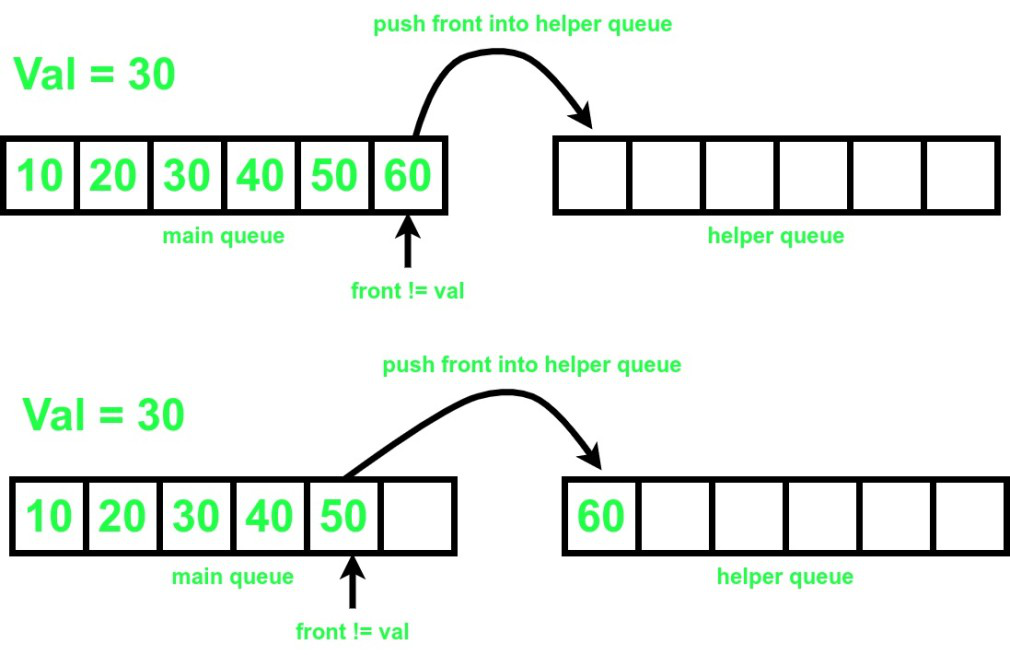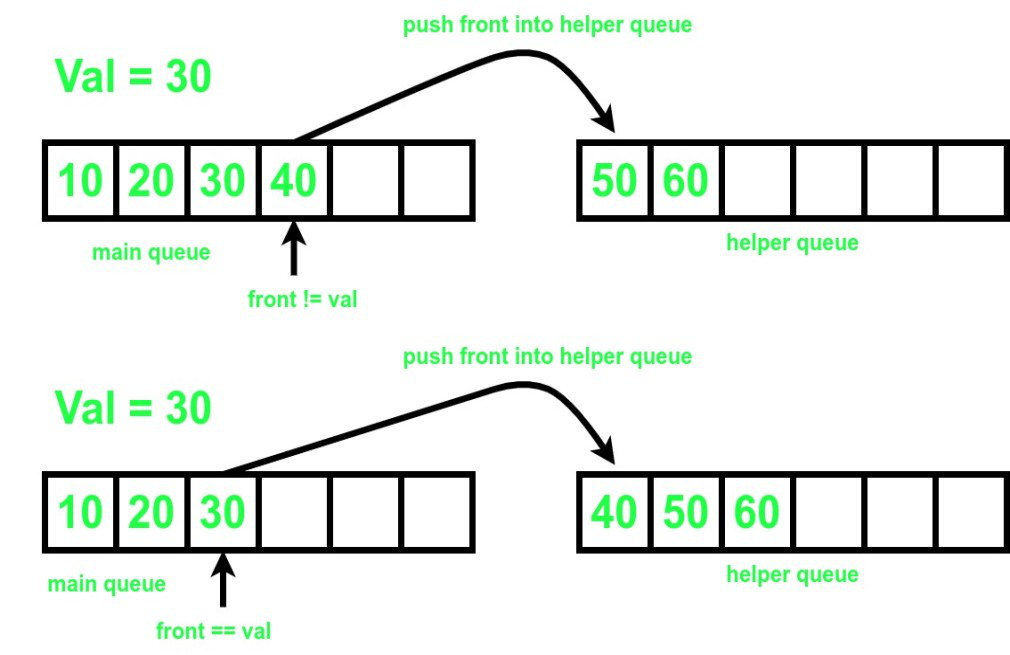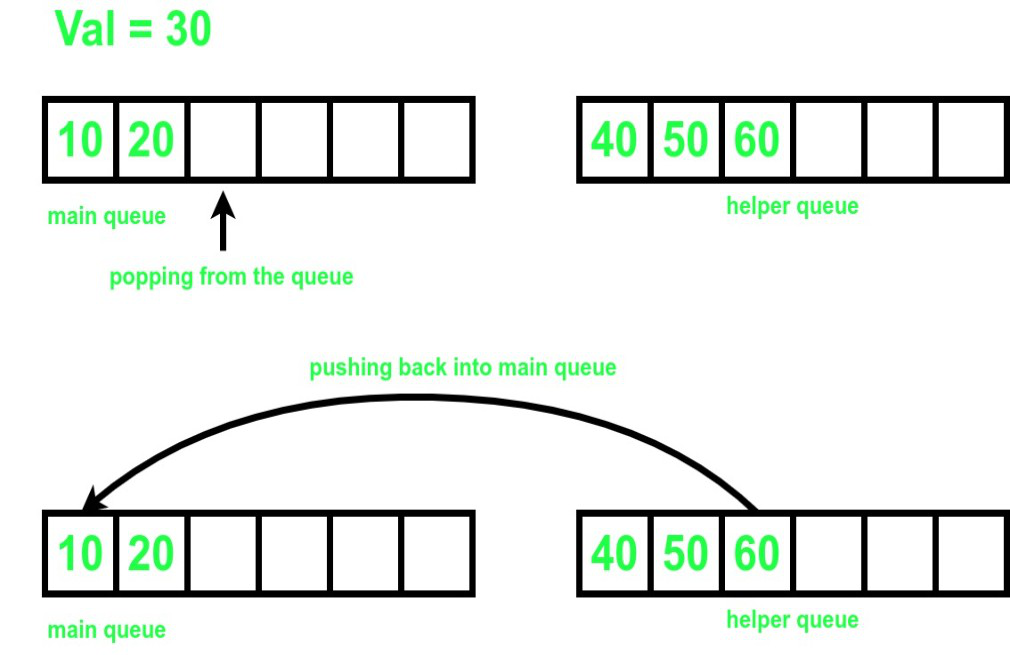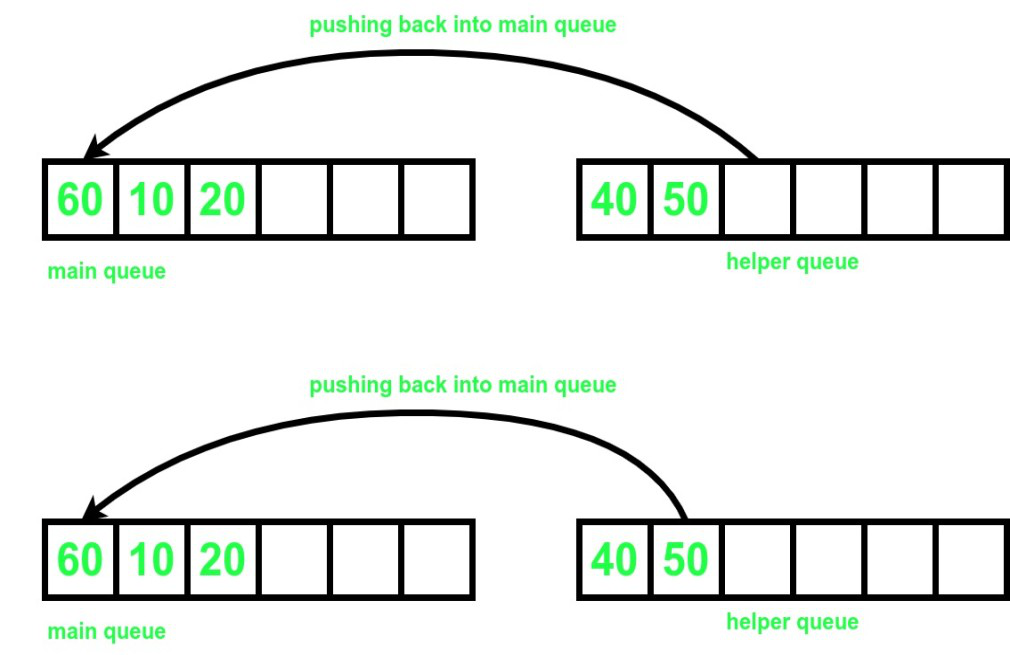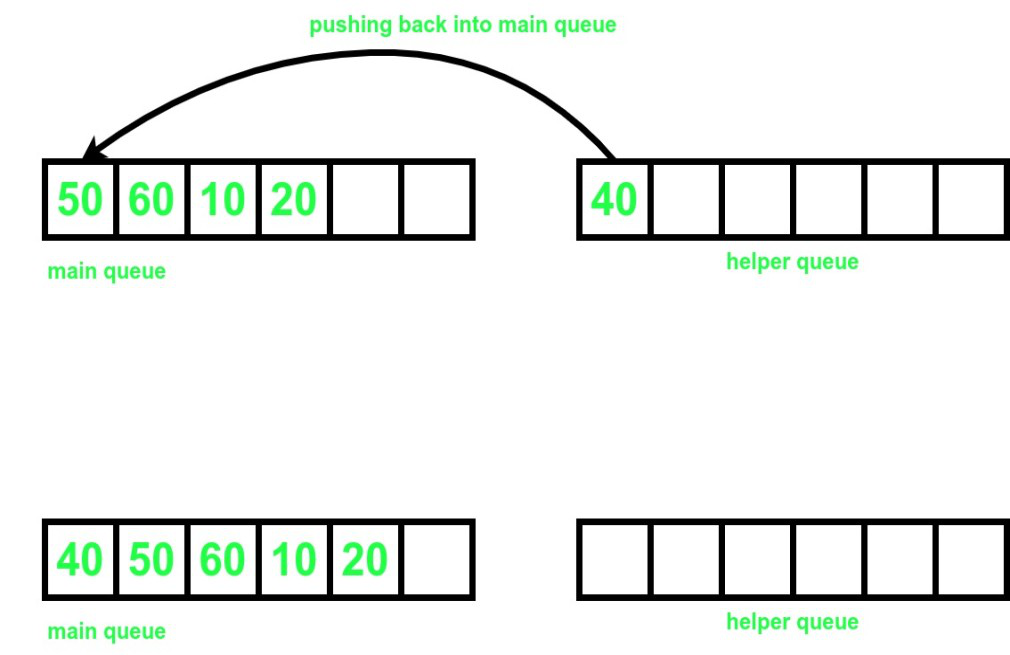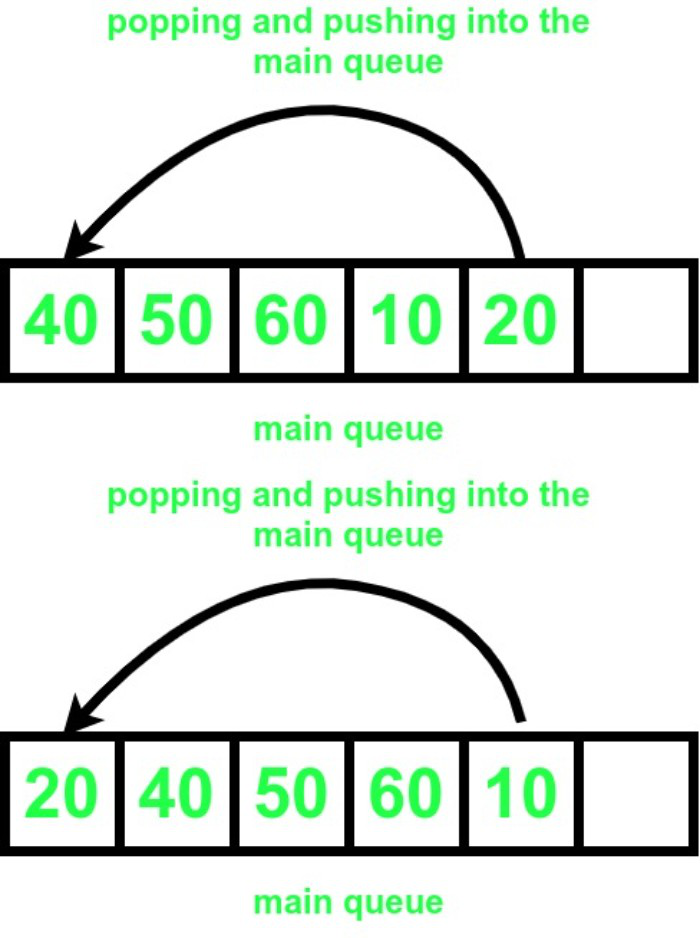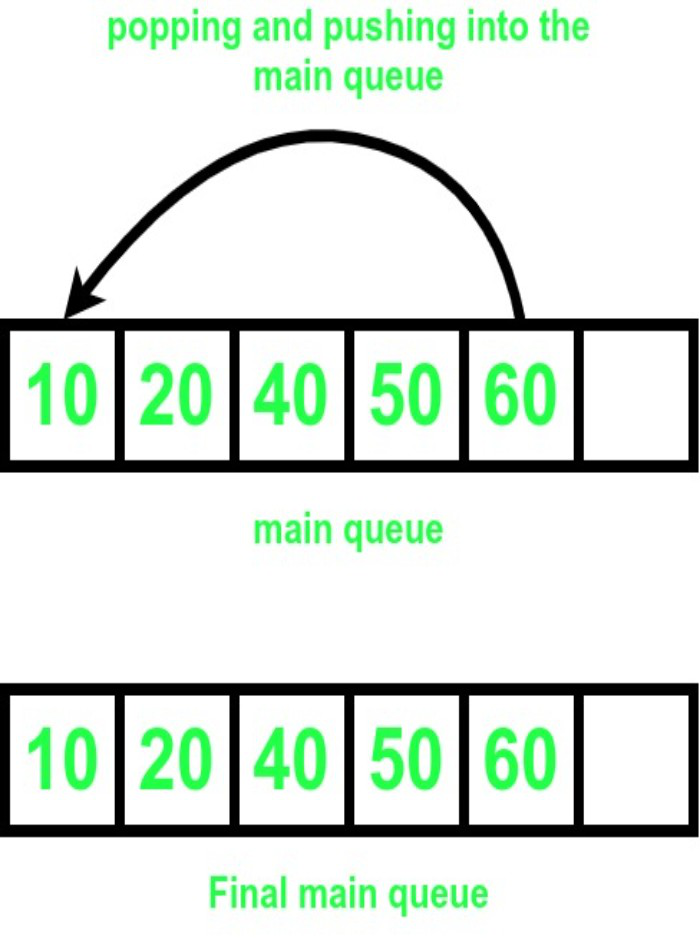Below is the implementation of the above approach.

## C++

 `// C++ program for the above approach.` `#include ` `using` `namespace` `std;`   `// Function to remove an element from` `// the queue` `void` `remove``(``int` `t, queue<``int``>& q)` `{`   `    ``// Helper queue to store the elements` `    ``// temporarily.` `    ``queue<``int``> ref;` `    ``int` `s = q.size();` `    ``int` `cnt = 0;`   `    ``// Finding the value to be removed` `    ``while` `(q.front() != t and !q.empty()) {` `        ``ref.push(q.front());` `        ``q.pop();` `        ``cnt++;` `    ``}`   `    ``// If element is not found` `    ``if` `(q.empty()) {` `        ``cout << ``"element not found!!"` `<< endl;` `        ``while` `(!ref.empty()) {`   `            ``// Pushing all the elements back into q` `            ``q.push(ref.front());` `            ``ref.pop();` `        ``}` `    ``}`   `    ``// If element is found` `    ``else` `{` `        ``q.pop();` `        ``while` `(!ref.empty()) {`   `            ``// Pushing all the elements back into q` `            ``q.push(ref.front());` `            ``ref.pop();` `        ``}` `        ``int` `k = s - cnt - 1;` `        ``while` `(k--) {`   `            ``// Pushing elements from front of q to its back` `            ``int` `p = q.front();` `            ``q.pop();` `            ``q.push(p);` `        ``}` `    ``}` `}`   `// Function to print all the elements` `// of the queue.` `void` `print(queue<``int``> qr)` `{` `    ``while` `(!qr.empty()) {` `        ``cout << qr.front() << ``" "``;` `        ``qr.pop();` `    ``}` `    ``cout << endl;` `}`   `// Driver Code` `int` `main()` `{` `    ``queue<``int``> q;`   `    ``// Pushing into the queue` `    ``q.push(10);` `    ``q.push(20);` `    ``q.push(30);` `    ``q.push(40);` `    ``q.push(50);` `    ``q.push(60);` `    ``print(q);`   `    ``// Removing 39 from the queue` `    ``remove``(39, q);` `    ``print(q);`   `    ``// Removing 30 from the queue` `    ``remove``(30, q);` `    ``print(q);` `    ``return` `0;` `}`

## Java

 `// Java program for the above approach.`   `import` `java.util.*;`   `class` `GFG{`   `// Function to remove an element from` `// the queue` `static` `Queue q;` `static` `void` `remove(``int` `t)` `{`   `    ``// Helper queue to store the elements` `    ``// temporarily.` `    ``Queue ref = ``new` `LinkedList<>();` `    ``int` `s = q.size();` `    ``int` `cnt = ``0``;`   `    ``// Finding the value to be removed` `    ``while` `(!q.isEmpty() && q.peek() != t) {` `        ``ref.add(q.peek());` `        ``q.remove();` `        ``cnt++;` `    ``}`   `    ``// If element is not found` `    ``if` `(q.isEmpty()) {` `        ``System.out.print(``"element not found!!"` `+``"\n"``);` `        ``while` `(!ref.isEmpty()) {`   `            ``// Pushing all the elements back into q` `            ``q.add(ref.peek());` `            ``ref.remove();` `        ``}` `    ``}`   `    ``// If element is found` `    ``else` `{` `        ``q.remove();` `        ``while` `(!ref.isEmpty()) {`   `            ``// Pushing all the elements back into q` `            ``q.add(ref.peek());` `            ``ref.remove();` `        ``}` `        ``int` `k = s - cnt - ``1``;` `        ``while` `(k-- >``0``) {`   `            ``// Pushing elements from front of q to its back` `            ``int` `p = q.peek();` `            ``q.remove();` `            ``q.add(p);` `        ``}` `    ``}` `}`   `// Function to print all the elements` `// of the queue.` `static` `void` `print()` `{` `    ``Queue qr = ``new` `LinkedList<>(q);` `    ``while` `(!qr.isEmpty()) {` `        ``System.out.print(qr.peek()+ ``" "``);` `        ``qr.remove();` `    ``}`   `    ``System.out.println();` `}`   `// Driver Code` `public` `static` `void` `main(String[] args)` `{` `    ``q = ``new` `LinkedList<>();`   `    ``// Pushing into the queue` `    ``q.add(``10``);` `    ``q.add(``20``);` `    ``q.add(``30``);` `    ``q.add(``40``);` `    ``q.add(``50``);` `    ``q.add(``60``);` `    ``print();`   `    ``// Removing 39 from the queue` `    ``remove(``39``);` `    ``print();`   `    ``// Removing 30 from the queue` `    ``remove(``30``);` `    ``print();` `}` `}`   `// This code is contributed by 29AjayKumar`

## C#

 `// C# program for the above approach.` `using` `System;` `using` `System.Collections;` `public` `class` `GFG{` `    `  `      ``// Function to remove an element from` `// the queue` `static` `Queue q = ``new` `Queue();` `static` `void` `remove_(``int` `t)` `{`   `    ``// Helper queue to store the elements` `    ``// temporarily.` `    ``Queue reff = ``new` `Queue();` `    ``int` `s = q.Count;` `    ``int` `cnt = 0;`   `    ``// Finding the value to be removed` `    ``while` `((``int``)q.Count != 0 && (``int``)q.Peek() != t) {` `      `  `        ``reff.Enqueue(q.Peek());` `        ``q.Dequeue();` `        ``cnt++;` `    ``}`   `    ``// If element is not found` `    ``if` `(q.Count == 0) {` `        ``Console.WriteLine(``"element not found!!"``);` `          `  `        ``while` `(reff.Count != 0) {` `            `  `            ``// Pushing all the elements back into q` `            ``q.Enqueue(reff.Peek());` `            ``reff.Dequeue();` `        ``}` `    ``}`   `    ``// If element is found` `    ``else` `{` `        ``q.Dequeue();` `        ``while` `(reff.Count != 0) {`   `            ``// Pushing all the elements back into q` `            ``q.Enqueue(reff.Peek());` `            ``reff.Dequeue();` `        ``}` `        ``int` `k = s - cnt - 1;` `        ``while` `(k-- >0) {`   `            ``// Pushing elements from front of q to its back` `            ``int` `p = (``int``)q.Peek();` `            ``q.Dequeue();` `            ``q.Enqueue(p);` `        ``}` `    ``}` `}`   `// Function to print all the elements` `// of the queue.` `static` `void` `print()` `{` `    ``Queue qr = (Queue)q.Clone(); ` `    ``while` `(qr.Count != 0) {` `        ``Console.Write(qr.Peek()+ ``" "``);` `        ``qr.Dequeue();` `    ``}`   `    ``Console.WriteLine();` `}`   `// Driver Code` `static` `public` `void` `Main (){`   `    ``// Pushing into the queue` `    ``q.Enqueue(10);` `    ``q.Enqueue(20);` `    ``q.Enqueue(30);` `    ``q.Enqueue(40);` `    ``q.Enqueue(50);` `    ``q.Enqueue(60);` `  `  `    ``print();`   `    ``// Removing 39 from the queue` `    ``remove_(39);` `    ``print();`   `    ``// Removing 30 from the queue` `    ``remove_(30);` `    ``print();` `}` `}`   `// This code is contributed by Dharanendra L V.`

Output

```10 20 30 40 50 60